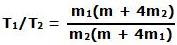## Wednesday, November 12, 2008

### Irodov Problem 1.246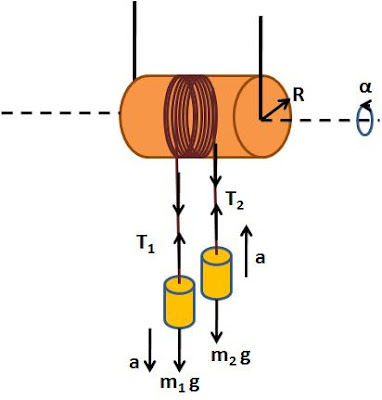Let the tensions in the string in the two sides of the cylindrical pulley connecting masses m1 and m2 be T1 and T2. The difference in tension is because of the friction between the string and the cylinder that is responsible for avoiding slippage and for turning the cylinder. Let the acceleration of the masses be a and let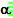be the angular acceleration of the cylinder. Let us assume that mass m1 is moving downwards and that mass m2 is moving upwards (the value of a will be negative if this is wrong).

Since the thread is not slipping over the cylinder,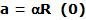There are two forces acting on mass m1, i) the tension in the string T1 pulling it upwards and ii) the force of gravity pulling it down. So we have,There are two forces acting on mass m2, i) the tension in the string T2 pulling it upwards and ii) the force of gravity pulling it down. So we have,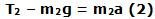Each of the tensions (T1 and T2) in the two parts of the string on either side of the cylinder induce a torque in the cylinder in opposite directions causing it to rotate. The moment of inertia of solid cylinder about an axis passing through its center parallel to its length is. Hence we have,Solving, for a from equations (1), (2) and (3) we obtain,Substituting (4) in (1) we get,Similarly substituting (4) in (2) we get,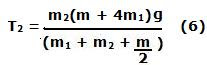From (5) and (6) we get,